What's new

# rand() Function in C Language

B

#### Bamdeb Ghosh

##### Guest
In the C language, the rand() function is used for Pseudo Number Generator(PRNG). The random numbers generated by the rand() function are not truly random. It is a sequence that repeats periodically, but the period is so large that we can ignore it. The rand() function works by remembering a seed value that is used to compute the next random number and the next new seed. In this article, we are going to discuss in detail how random numbers can be generated using the rand() function. So, let’s get started!

Header File:

stdlib.h

Syntax:

int rand (void)

Return values:

This function returns the next pseudo-random number in the series. The range value of the number series is between 0 and RAND_MAX. RAND_MAX is a macro defined in stdlib.h header file, whose value is the maximum value, which can return by rand() function. The value of RAND_MAX is greater but not less than 32767 depending on the C libraries.

//Example1.c

#include
#include

int main()
{

int i;

printf("10 Random Numbers =>\n");

for(i=0;i<10;i++)
{
printf("%d ",rand());
}

printf("\n");
return 0;
}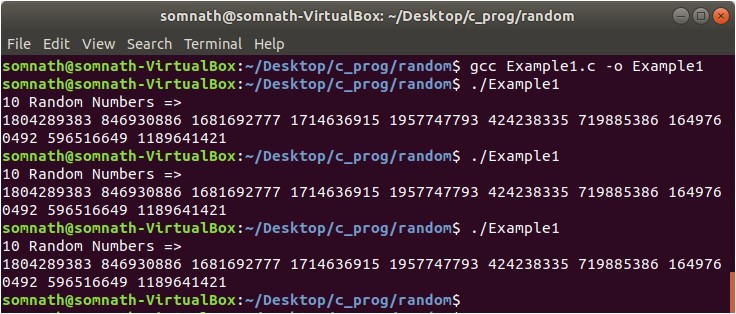In Example1.c, we call the rand() function in each iteration of for loop and print the return value of the function. The value sequence of the rand() function is the same each time we run the program. By default, the seed of the rand function is set to 1.

We can set the seed for the rand function using the srand() function. The seed can be set only once, and before the first time rand() function call.

srand() function:

Header File:

stdlib.h

Syntax:

int srand (unsigned int seed)

Arguments:

This function takes 1 argument

seed: An integer value used as a seed for a new series of pseudo-random numbers.

Return values:

None

//Example2.c

#include
#include
#include

int main()
{

int i;

srand(time(0));

printf("10 Random Numbers =>\n");

for(i=0;i<10;i++)
{
printf("%d ",rand());
}

printf("\n");
return 0;
}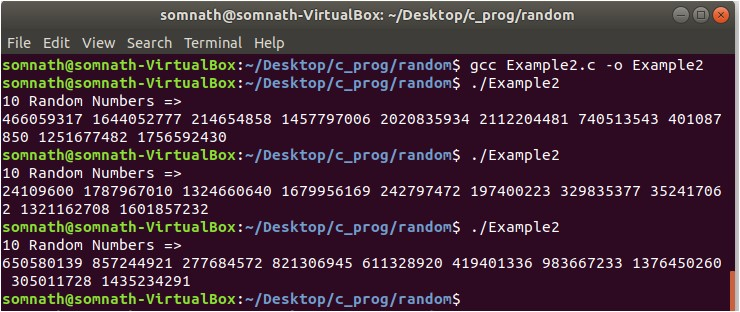In Example2.c, we have used the srand() function to set the initial seed of the random number sequence generated by rand() function. Each time the program is run, a different sequence is generated. In srand(), time(0) function (declared in time.h header file) is used as a seed. This time(0) function returns the number of seconds elapsed since the epoch (00:00:00, January 1, 1970). This still may produce the same sequences if you run the program in the same second.

//Example3.c

#include
#include
#include

int main()
{

int i;

srand(time(0));

printf("10 Random Numbers between 1 and 10=>\n");

for(i=0;i<10;i++)
{
printf("%d ",(rand() %10) + 1);
}

printf("\n");
return 0;
}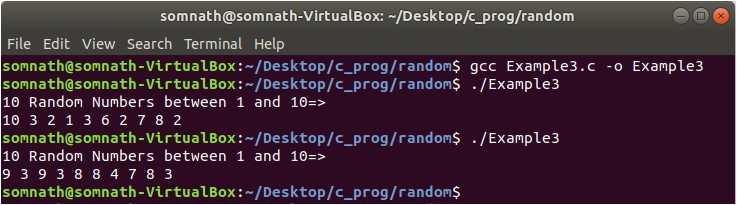In Example3.c we have seen how random numbers can be generated between 1 and 10.

//Example4.c

#include
#include
#include

int main()
{

int i,max,min;

printf("Enter Min value => ");
scanf("%d",&min);
printf("Enter Max value => ");
scanf("%d",&max);

if(min>max)
{
printf("Min value is greater than max value\n");
return 0;
}

srand(time(0));

printf("10 Random Numbers between %d and %d=>\n",min,max);

for(i=0;i<10;i++)
{
printf("%d ",(rand() % (max - min +1)) + min);
}

printf("\n");
return 0;
}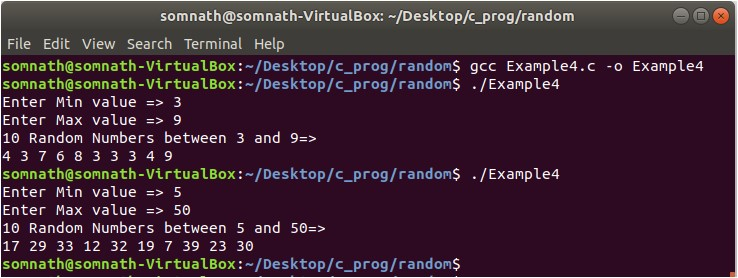In Example4.c we have taken the range from the user and generated a random number within this range. The formula is: rand() % (max – min +1)) + min

//Example5.c

#include
#include
#include

int main()
{

int i;

srand(time(0));

printf("10 Random Numbers between 0.0 and 1.0=>\n");

for(i=0;i<10;i++)
{
printf("%f ",((float)rand() /RAND_MAX));
}

printf("\n");
return 0;
}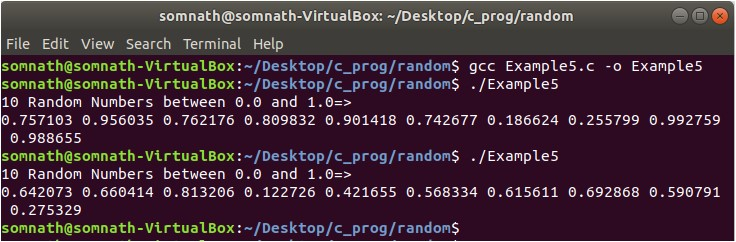In Example5.c, we have seen how we can generate random numbers between float 0.0 and 1.0 The formula is: (float)rand() /RAND_MAX)

//Example6.c

#include
#include
#include

int main()
{

int i;
float max,min;

printf("Enter Min value => ");
scanf("%f",&min);
printf("Enter Max value => ");
scanf("%f",&max);

if(min>max)
{
printf("Min value is greater than max value\n");
return 0;
}

srand(time(0));

printf("10 Random Numbers between %f and %f =>\n",min,max);

for(i=0;i<10;i++)
{
printf("%f ",min + ((float)rand() /(RAND_MAX/(max - min))));
}

printf("\n");
return 0;
}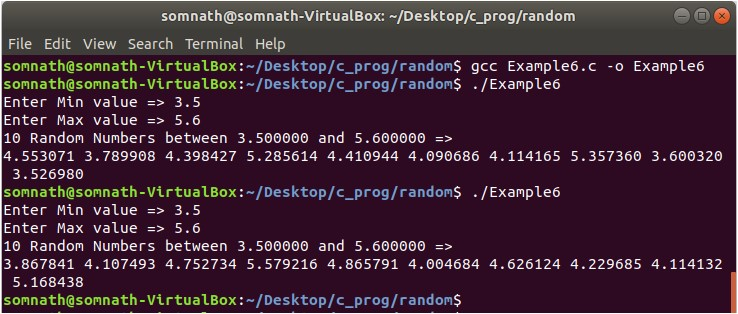In Example6.c, we have taken the range from the user and generated a random number within this range (both inclusive). The formula is: min + ((float)rand() /(RAND_MAX/(max – min)))

Conclusion:

In this article, we have learned how random numbers can be generated using the rand() and srand() function. There are no guarantees about the quality of the random numbers generated by the rand function, but it is good enough for casual use.

Sources:

n.d., (2008, October 14). Purpose of the GNU C Library.. Retrieved from The GNU C Library - 19. Mathematics

Matsumoto, Y. (2001, November). Ruby in a Nutshell. Retrieved from Download Limit Exceeded

Credit to linux